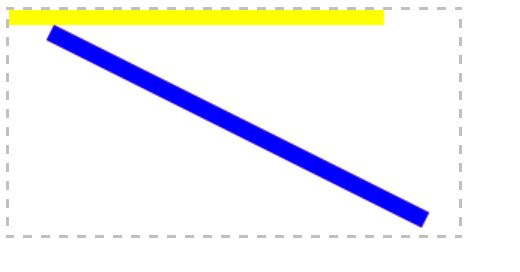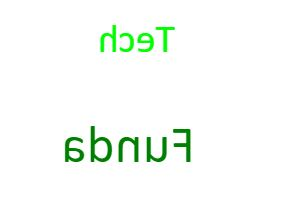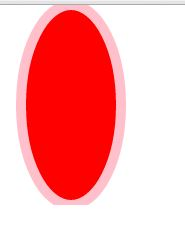# Canvas Transformations in HTML5

### Transformations

Transformations are used to create different transforms in the webpage, They are different types of transformations they are translate, rotate, transform etc

### Translate()

Translate is used to change the positions of the given canvas by using` translate(x,y)`  method which is used to move the canvas and its origin to a different point in the grid. Here argument x is the amount the canvas is moved to the `left or right`, and y is the amount it's moved `up or down`

```  <canvas id="myCanvas" width="200" height="150" style="border:5px dotted green;">
</canvas>

<script>

var a = document.getElementById("myCanvas");
var b = a.getContext("2d");

b.fillStyle = "yellow";
b.fillRect(10, 10, 100, 100);

b.translate(40, 30);

b.fillStyle = "blue";
b.fillRect(10, 10, 100, 100);

</script>```

In the above code snippe we have defined the translate. We have taken canvas as "mycanvas", width as 200 and height as 150.

• b.fillRect(10, 10, 100, 100); - It renders the (x, y width, height) positions of the rectangle with fillstyle color as yellow
• `b.translate(40, 30);` - It is used to change the (x, y) positions of the canvas
• b.fillRect(10, 10, 100, 100); -It renders the (x, y width, height) positions of the rectangle with fillstyle color as blue

output### Scaling()

Scaling uses the method `scale(x,y)` method to increase or decrease the units in canvas grid, This is used to draw the shapes. This method takes two parameters where x is the scale factor in the `horizontal` direction and y is the scale factor in the `vertical` direction. Both parameters must be positive numbers.

```<canvas id="myCanvas" width="500" height="200"></canvas>
<script>
var canvas = document.getElementById('myCanvas');
var context = canvas.getContext('2d');
var rectWidth = 14;
var rectHeight = 12;

context.scale(12, 16);
context.fillStyle = 'orange';
context.fillRect(7, 6, 14, 12);
</script>```

Scaling

In the above code snippe we have defined the translate. We have taken canvas as "mycanvas", width as 500 and height as 200.

We have taken the rectangle (width, height) as (14, 12).

• `context.scale(12, 16)` as to set the positions of the rectangle
• context.fillstyle as the color of orange
• context.fillrect(7,6,14,12) as the values of the rectangle

output### Transform()

Transformation method consists of the

• transform()
• settransform()

### Transform method()

This method changes the transformation matrix to apply the matrix given by the arguments.
``` <canvas `id="myCanvas"` width="300" height="200" style="border:3px dotted silver;">
Your browser does not support the HTML5 canvas tag.
</canvas>

<script>

var a = document.getElementById("myCanvas");
var b = a.getContext("2d");

b.fillStyle = "orange";
b.fillRect(0, 0, 250, 10)

b.transform(1, 0.5, -0.5, 1, 3, 1);
b.fillStyle = "silver";
b.fillRect(0, 0, 250, 10);

b.transform(1, 0.5, 0, 1, 0, 10);
b.fillStyle = "green";
b.fillRect(0, 0, 250, 10);

</script>
```

Transform

In the above code snipet we have defined the transform method we have three rectangles with width and height with fill styles colors as green, silver, orange

• `b.transform(1, 0.5, -0.5, 1, 3, 1);` - It renders the transforming values of the rectangle
• b.fillStyle = "silver"; - It renders the color of the rectangle
• b.fillRect(0, 0, 250, 10); - It renders the size of the rectangle

output### SetTransform()

This method changes the transformation matrix to the matrix given by the arguments . This transform passes the values of the same arguments to the value

``` <canvas id="myCanvas" width="300" height="150" style="border:2px dashed silver;">
Your browser does not support the HTML5 canvas tag.
</canvas>

<script>

var a = document.getElementById("myCanvas");
var b = a.getContext("2d");

b.fillStyle = "yellow";
b.fillRect(0, 0, 250, 10)

b.setTransform(1, 0.5, -0.5, 1, 30, 10);
b.fillStyle = "red";
b.fillRect(0, 0, 250, 10);

b.setTransform(1, 0.5, -0.5, 1, 30, 10);
b.fillStyle = "blue";
b.fillRect(0, 0, 250, 10);

</script>
```

SetTransform()

In the above code snipet we have defined the set transform method we have three rectangles with width and height with fill stylesblue, red, yellow, It resets the current transforms and exists with the same argument values

• `b.transform(1, 0.5, -0.5, 1, 3, 1); `- It renders the transforming values of the rectangle
• b.fillStyle = "silver"; - It renders the color of the rectangle
• b.fillRect(0, 0, 250, 10); - It renders the size of the rectangle

output### Shear transform

The transform() method is used to shear the canvas. the shear transform uses the matrix method, The horizontal shear is defined by sx and the vertical shear is defined by sy.

The shear matrix is defined as

```         1  sx  0
sy  1  0
0  0  1
```

The horizontal shear is defined by sx and the vertical shear is defined by sy.

```    <canvas id="myCanvas" width="400" height="200" style="border:2px solid green"></canvas>
<script>
var a = document.getElementById('myCanvas');
var b = a.getContext('2d');
var rectWidth = 150;
var rectHeight = 75;

// shear matrix:
//  1  sx  0
//  sy  1  0
//  0  0  1

var sx = 0;
// .75 horizontal shear
var sy = 1;
// no vertical shear
b.transform(1, sx, sy, 1, 0, 0);

b.fillStyle = 'orange';
b.fillRect(30, 50, 150, 75);
</script>
```

Shear transform()

In the above code snippet we have defined the shear which uses the matrix form to create the shear transform.

We have canvas width as 400 and height as 200 and style as border as 2px solid green;

• var a = document.getElementById('myCanvas'); -  we have declared var a as document .getElementbyid as "mycanvas"
• var b = a.get context("2d"); and rectangle width as 150 and height as 75 and we have the shear matrix as we defined the shear matrix sx value as 0 and sy value as 1.
• b.transform() method is used to define the shear matrix by using the` (1, sx, sy, 1,0,0);-` deffines the value with fillstyle color as orange
• b.fillrect as (x, y, width, height) as (30, 50, 150, 75); - defines the rectangle values

outputMirror in horizontal form

In order to use the mirror we need to use the scale as negative

The syntax for the negative scale is `scale(-1,2)`

``` <canvas id="myCanvas" width="500" height="200"></canvas>
<script>
var canvas = document.getElementById('myCanvas');
var context = canvas.getContext('2d');

context.translate(250, 50);
context.scale(-1, 1);
context.font = '30pt Calibri';
context.fillStyle = 'lime';
context.fillText('Tech', 50, 20);

context.translate(-70, 50);
context.font = '40pt Calibri';
context.fillStyle = 'green';
context.fillText('Funda', 100, 80);
</script>
```

Mirror in horizontal

In the above code snippet we have defined the mirror to display in horizontal form we need to use the `scale as (-1,1).` we have two texts with different values to display the value in mirror as reverse

• context.translate(250, 50); - It is used to display the text in the center of the canvas
• `context.scale(-1, 1);` - It is used to define the mirror in the horizontal form
• context.font = '30pt Calibri'; - It is used to increase the fontsize of the text
• context.fillStyle = 'lime'; - It is used to apply the text with color
• context.fillText('Tech', 50, 20); - It is used to fill the text and values of the (x,y) positions

output### Mirror to diplay in the vertical form

It is used to display the text in the vertical form

The syntax for the displaying of the mirror in vertical form is scale(1,-1)

```  <canvas id="myCanvas" width="500" height="200"></canvas>
<script>
var canvas = document.getElementById('myCanvas');
var context = canvas.getContext('2d');

context.translate(150, 50);
context.scale(1, -1);
context.font = '30pt Calibri';
context.fillStyle = 'blue';
context.fillText('DOTNETFUNDA', 50, 20);
</script>
```

Mirror in vertical form

In the above code snippet we have defined the mirror to display in vertical form we need to use the scale as (1,-1). we have text with different values to display the value in mirror as reverse

• context.translate(150, 50); - It is used to display the text in the center of the canvas
• `context.scale(1, -1); `- It is used to define the mirror in the horizontal form
• context.font = '30pt Calibri'; - It is used to increase the fontsize of the text
• context.fillStyle = 'blue'; - It is used to apply the text with color
• context.fillText('DOTNETFUNDA', 50, 20); - It is used to fill the text and values of the (x,y) positions

output### Oval

It defines the shape of the canvas in oval

```<canvas id="myCanvas" width="600" height="200"></canvas>
<script>
var canvas = document.getElementById('myCanvas');
var context = canvas.getContext('2d');
var centerX = 0;
var centerY = 0;

context.save();
context.translate(300, 100);
context.scale(1, 2);
context.beginPath();
context.arc(0, 0, 50, 0, 2* Math.PI, false);

context.restore();
context.fillStyle = 'red';
context.fill();
context.lineWidth = 10;
context.strokeStyle = 'pink';
context.stroke();
</script>
```

Oval

In the above code snippet we have defined  the how to create the oval we have taken the center as (x, y) and radius as 50

• context.save(); - It is used to push the current context on to the stack
• context.translate(300, 100); - It is used to translate the into the center of the canvas
• context.scale(1, 2); - It is used to scale the drawings bigger and smaller
• context.arc(0, 0, 50, 0, 2* Math.PI, false); - It is used to draw the arc with given values
• context.fillStyle = 'red'; - It is used to fill the center of the color with red
• context.lineWidth = 10; - It is used to add the line width of size 10
• context.strokeStyle = 'pink'; - It is used to add the color to the linewidth  as pink

outputViews: 3238 | Post Order: 35